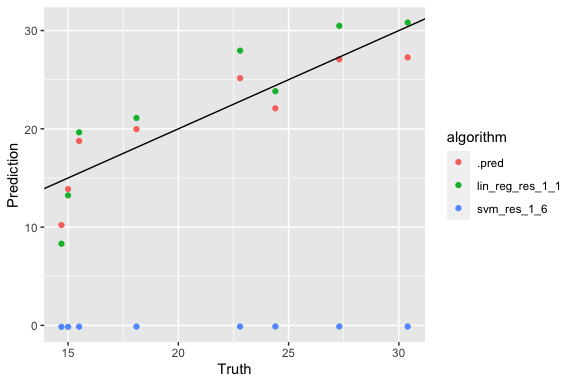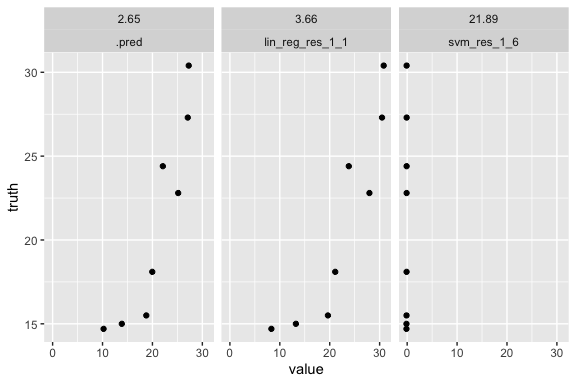# Regression Example

library(ensModelVis)

An example of fitting a stacked regression ensemble from stacks package vignette and using ensModelVis for visualising the models.

Packages we will need:

library(tidymodels)
library(stacks)

Dataset: predict mpg based on other attributes in mtcars data.

data("mtcars")
mtcars <- mtcars %>% mutate(cyl = as.factor(cyl), vs = as.factor(vs), am = as.factor(am))

Split the training data, generate resamples, set the recipe and metric.


set.seed(1)
mtcars_split <- initial_split(mtcars)
mtcars_train <- training(mtcars_split)
mtcars_test  <- testing(mtcars_split)

set.seed(1)
folds <- vfold_cv(mtcars_train, v = 5)

mtcars_rec <-
recipe(mpg ~ .,
data = mtcars_train)

metric <- metric_set(rmse)

ctrl_grid <- control_stack_grid()
ctrl_res <- control_stack_resamples()

Fit a linear model and a support vector machine model (with hyperparameters to tune).

# LINEAR REG
lin_reg_spec <-
linear_reg() %>%
set_engine("lm")

# extend the recipe
lin_reg_rec <-
mtcars_rec %>%
step_dummy(all_nominal())

# add both to a workflow
lin_reg_wflow <-
workflow() %>%

# fit to the 5-fold cv
set.seed(2020)
lin_reg_res <-
fit_resamples(
lin_reg_wflow,
resamples = folds,
metrics = metric,
control = ctrl_res
)

# SVM
svm_spec <-
svm_rbf(
cost = tune("cost"),
rbf_sigma = tune("sigma")
) %>%
set_engine("kernlab") %>%
set_mode("regression")

# extend the recipe
svm_rec <-
mtcars_rec %>%
step_dummy(all_nominal()) %>%
step_impute_mean(all_numeric(), skip = TRUE) %>%
step_corr(all_predictors(), skip = TRUE) %>%
step_normalize(all_numeric(), skip = TRUE)

# add both to a workflow
svm_wflow <-
workflow() %>%

# tune cost and sigma and fit to the 5-fold cv
set.seed(2020)
svm_res <-
tune_grid(
svm_wflow,
resamples = folds,
grid = 6,
metrics = metric,
control = ctrl_grid
)

Use stacks to get the ensemble:

mtcars_model_st <-
stacks() %>%
blend_predictions() %>%
fit_members()

Predict with test data:

member_preds <-
mtcars_test %>%
select(mpg) %>%
bind_cols(predict(mtcars_model_st, mtcars_test, members = TRUE))

Evaluate RMSE from each model (Stacking decreases RMSE):

map_dfr(member_preds, rmse, truth = mpg, data = member_preds) %>%
mutate(member = colnames(member_preds))
#> # A tibble: 4 × 4
#>   .metric .estimator .estimate member
#>   <chr>   <chr>          <dbl> <chr>
#> 1 rmse    standard        0    mpg
#> 2 rmse    standard        2.65 .pred
#> 3 rmse    standard        3.66 lin_reg_res_1_1
#> 4 rmse    standard       21.9  svm_res_1_6

SVM does not make useful predictions here. We can see this from the RMSE and more clearly from the plots:

p1 <- plot_ensemble(truth = member_preds$mpg, tibble_pred = member_preds %>% select(-mpg)) p1 + geom_abline()plot_ensemble(truth = member_preds$mpg, tibble_pred = member_preds %>% select(-mpg), facet = TRUE)
#> Joining, by = "name"